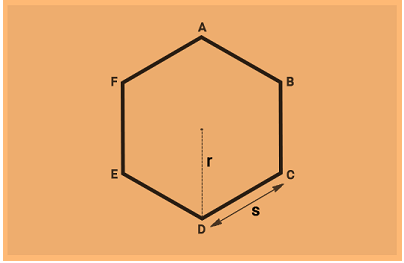# Area of a Hexagon Formula

A polygon with six sides and six interior angles is known as hexagon. Like squares and equilateral triangles, regular hexagons fit together without any gaps to tile the plane. A regular hexagon resembles a convex figure with sides of the same length, and comprises of internal angles of 120 degrees. It also has six rotational symmetries and six reflection symmetries. Hexagons are the only regular polygon that can be subdivided into another regular polygon. Hexagons are the first polygons – when ascending by number of sides – that do not form the faces of a regular convex polyhedron in Euclidean space. Hexagons are third-order permutohedron, meaning each vertex of a hexagon can be described with Cartesian coordinates using one of six permutations of the numbers 1, 2, and 3. The area of hexagon is determined using the side and radius of it i.e. s and r.The Area of a Hexagon Formula is =  A = 3sr
Where,
s is the side of the hexagon.
r is the radius of the hexagon.

### Solved Examples

Question 1: Find the area of a hexagon whose side is 4 cm and radius is 6 cm ?
Solution:
Given,
s = 4 cm
r = 6 cm
Area of a hexagon
= 3 $\times$ s $\times$ r
= 3 $\times$ 4 $\times$ 6 cm2
= 72 cm2